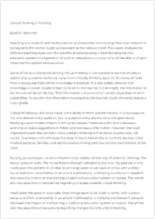# Atwood’s Machine Experiment

Available Only on StudyHippo
Topics:
• Pages: 1 (268 words)
• Published: November 30, 2017
Text preview

Carleton University
Laboratory ReportAtwood’s Machine ExperimentCourse

Number: PHYS 1007

Experiment Number: 3Lab

Period: Thursday

AMGroup Number: L1

Lab Partner: Zain Amer
Workstation Number: 16

Performed: October 10, 2013
Date Submitted: October 24, 2013

This Report Submitted by: Ryan Maxwell

Purpose: The purpose of this experiment was to measure the acceleration due to gravity (g) using Atwood’s Machine, and to also measure the frictional torque of the machine.

Theory: Assuming that m2 is greater than m1, and applying Newton’s second law of motion on these two masses gives two equations:m2g-T2=m2a

1. T1-m1g=m1a
2. For rotating systems, friction always shows up in the form of torque for equations of motion, which slow
...

s the rotation. There is a counter-clockwise torque that exists:?=T2’r-T1’r

3. where T1’ and T2’ have the same magnitudes as T1 and T2 and are the reactions of these two terms. The term r represents the radius of the pulley. The torque causes the pulley to accelerate angularly, which is given the symbol ?. The rotation of the pulley is then the torque equation: I?=T2r-T1r-?
4. where I am the rotational inertia of the pulley, and ? is the torque caused by the friction on the axel. It can be assumed that the angle between the string and the radius of the pulley is 90o. In this way the sin(?) = 1.
Assuming that there is no slipping of the string over the pulley, one can solve for one of the four unknowns remaining, ?:?=ar
5. When one substitutes this equation into the torque equation for ?, the result is Iar2+?r=T2-T1.

Join StudyHippo to see entire essay
Join StudyHippo to see entire essay